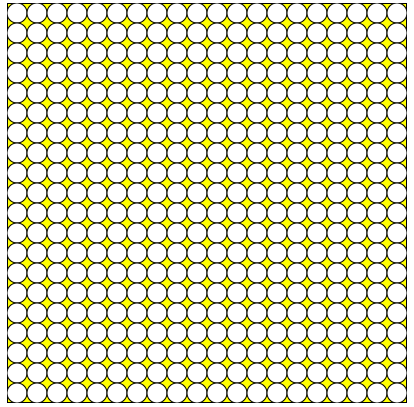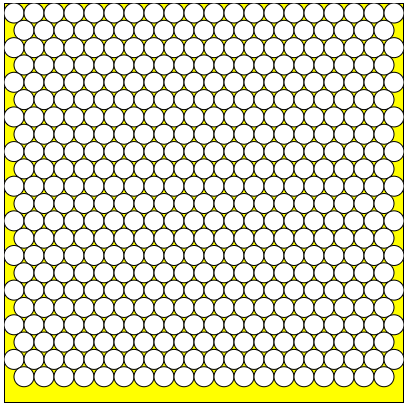Engineering ToolBox - Resources, Tools and Basic Information for Engineering and Design of Technical Applications!

# Circles within a Rectangle - Calculator

## Calculate the maximum number of circles within a rectangle - can be used to calculate the numbers of pipes or wires in a conduit or similar.

The calculator below estimates the maximum number of circles that may fit within a rectangle. The calculator can be used to calculate

• the number of pipes - or wires - that fits within a conduit or similar applications

Input the rectangle inside dimensions - height and width and the circles outside diameters.

Default values are for 0.5 inch circles inside a 10 inch x 10 inch square. The calculator is generic and any kind of units can be used - as long as the same units are used for all values.

### Rectangular Pattern#### Rectangular Pattern - Circle Coordinates

Copy and paste the circle center coordinates to your application. x = 0 and y = 0 is top left corner of rectangle.

x y

Tip! - the values can be adapted and modified in excel or in a text editor for use in a CNC G-code generator or similar.

### Triangular Pattern#### Triangular Pattern - Circle Coordinates

Copy and paste the circle center coordinates to your application. x = 0 and y = 0 is top left corner of rectangle.

x y

Be aware! - The algorithm is quite simple - switching rectangle width and height may influence the number calculated. Switching the input values above changes the layout and gives

Note! - with some combinations of rectangular shapes and circle sizes - one or two more circles - or even more - may be added with a modified layout of the circles. In the default triangular example above - two more circles can be added in between if the left and right bottom circles are moved to the left and right border. The algorithm used for the calculation is quite simple and may underestimate the number of circles in some cases.

## Related Topics

• ### Electrical

Electrical units, amps and electrical wiring, wire gauge and AWG, electrical formulas and motors.
• ### Mathematics

Mathematical rules and laws - numbers, areas, volumes, exponents, trigonometric functions and more.

## Related Documents

• ### Area of Intersecting Circles

Calculate area of intersecting circles
• ### Area Survey App

Online app that can be used to make an exact plot of a surveyed area - like a room, a property or any 2D shape.
• ### Area Units Converter

Convert between units of area.
• ### Circles - Circumferences and Areas

Circumferences and areas of circles with diameters in inches.
• ### Circles Outside a Circle

Calculate the numbers of circles on the outside of an inner circle - like the geometry of rollers on a shaft.
• ### Discrete Data Sets and their Mean, Median and Mode Values

Calculate the arithmetic mean, median and modal values from discrete data sets.
• ### Elementary Curves

Ellipse, circle, hyperbola, parabola, parallel, intersecting and coincident lines.
• ### Elementary Surfaces

Ellipsoid, sphere, hyperboloid, cone and more.
• ### Equal Areas - Circles vs. Squares

Radius and side lengths of equal areas, circles and squares.
• ### Smaller Circles within a Large Circle - Calculator

Calculate the number of small circles that fits into an outer larger circle - ex. how many pipes or wires fits into a larger pipe or conduit.
• ### Smaller Rectangles within a Larger Rectangle

The maximum number of smaller rectangles - or squares - within a larger rectangle (or square).
• ### Solving Equations of the First Degree with One Unknown

Equations with one unknown.
• ### Solving First Degree Equations with Two Unknowns

Online calculator and solbing procedure for two equations with two unknowns.
• ### Solving Quadratic Equation with One Unknown

How to solve a quadratic equation.
• ### Squaring with Diagonal Measurements

A rectangle is square if the lengths of both diagonals are equal.

## Engineering ToolBox - SketchUp Extension - Online 3D modeling!

Add standard and customized parametric components - like flange beams, lumbers, piping, stairs and more - to your Sketchup model with the Engineering ToolBox - SketchUp Extension - enabled for use with older versions of the amazing SketchUp Make and the newer "up to date" SketchUp Pro . Add the Engineering ToolBox extension to your SketchUp Make/Pro from the Extension Warehouse !

We don't collect information from our users. More about

## Citation

• The Engineering ToolBox (2014). Circles within a Rectangle - Calculator. [online] Available at: https://www.engineeringtoolbox.com/circles-within-rectangle-d_1905.html [Accessed Day Month Year].

Modify the access date according your visit.

9.27.11